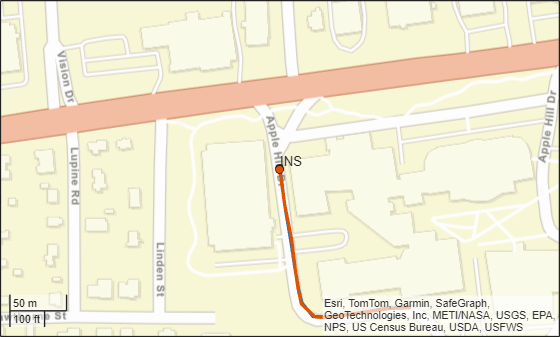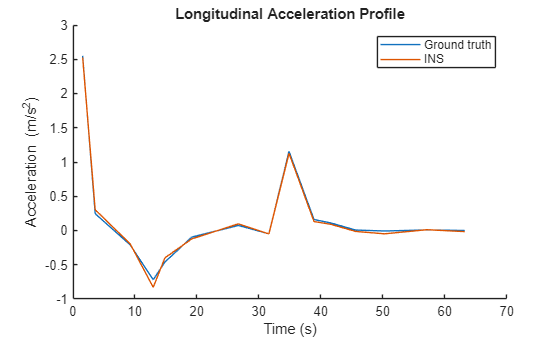# state

Inertial ground-truth state of actor

## Syntax

``gTruth = state(ac)``

## Description

example

````gTruth = state(ac)` returns the inertial ground-truth state of an actor. Use this data as the input ground truth for an `insSensor` System object™.This function is supported only for actors that have trajectories created by using the `smoothTrajectory` function.```

## Examples

collapse all

Generate measurements from an INS sensor that is mounted to a vehicle in a driving scenario. Plot the INS measurements against the ground truth state of the vehicle and visualize the velocity and acceleration profile of the vehicle.

Create Driving Scenario

Load the geographic data for a driving route at the MathWorks® Apple Hill campus in Natick, MA.

```data = load('ahroute.mat'); latIn = data.latitude; lonIn = data.longitude; ```

Convert the latitude and longitude coordinates of the route to Cartesian coordinates. Set the origin to the first coordinate in the driving route. For simplicity, assume an altitude of 0 for the route.

```alt = 0; origin = [latIn(1),lonIn(1),alt]; [xEast,yNorth,zUp] = latlon2local(latIn,lonIn,alt,origin); ```

Create a driving scenario. Set the origin of the converted route as the geographic reference point.

```scenario = drivingScenario('GeoReference',origin); ```

Create a road based on the Cartesian coordinates of the route.

```roadCenters = [xEast,yNorth,zUp]; road(scenario,roadCenters); ```

Create a vehicle that follows the center line of the road. The vehicle travels between 4 and 5 meters per second (9 to 11 miles per hour), slowing down at the curves in the road. To create the trajectory, use the `smoothTrajectory` function. The computed trajectory minimizes jerk and avoids discontinuities in acceleration, which is a requirement for modeling INS sensors.

```egoVehicle = vehicle(scenario,'ClassID',1); egoPath = roadCenters; egoSpeed = [5 5 5 4 4 4 5 4 4 4 4 5 5 5 5 5]; smoothTrajectory(egoVehicle,egoPath,egoSpeed); ```

Plot the scenario and show a 3-D view from behind the ego vehicle.

```plot(scenario) chasePlot(egoVehicle) ```Create INS Sensor

Create an INS sensor that accepts the input of simulation times. Introduce noise into the sensor measurements by setting the standard deviation of velocity and accuracy measurements to 0.1 and 0.05, respectively.

```INS = insSensor('TimeInput',true, ... 'VelocityAccuracy',0.1, ... 'AccelerationAccuracy',0.05); ```

Visualize INS Measurements

Initialize a geographic player for displaying the INS measurements and the actor ground truth. Configure the player to display its last 10 positions and set the zoom level to 17.

```zoomLevel = 17; player = geoplayer(latIn(1),lonIn(1),zoomLevel, ... 'HistoryDepth',10,'HistoryStyle','line'); ```Pre-allocate space for the simulation times, velocity measurements, and acceleration measurements that are captured during simulation.

```numWaypoints = length(latIn); times = zeros(numWaypoints,1); gTruthVelocities = zeros(numWaypoints,1); gTruthAccelerations = zeros(numWaypoints,1); sensorVelocities = zeros(numWaypoints,1); sensorAccelerations = zeros(numWaypoints,1); ```

Simulate the scenario. During the simulation loop, obtain the ground truth state of the ego vehicle and an INS measurement of that state. Convert these readings to geographic coordinates, and at each waypoint, visualize the ground truth and INS readings on the geographic player. Also capture the velocity and acceleration data for plotting the velocity and acceleration profiles.

```nextWaypoint = 2; while advance(scenario) % Obtain ground truth state of ego vehicle. gTruth = state(egoVehicle); % Obtain INS sensor measurement. measurement = INS(gTruth,scenario.SimulationTime); % Convert readings to geographic coordinates. [latOut,lonOut] = local2latlon(measurement.Position(1), ... measurement.Position(2), ... measurement.Position(3),origin); % Plot differences between ground truth locations and locations reported by sensor. reachedWaypoint = sum(abs(roadCenters(nextWaypoint,:) - gTruth.Position)) < 1; if reachedWaypoint plotPosition(player,latIn(nextWaypoint),lonIn(nextWaypoint),'TrackID',1) plotPosition(player,latOut,lonOut,'TrackID',2,'Label','INS') % Capture simulation times, velocities, and accelerations. times(nextWaypoint,1) = scenario.SimulationTime; gTruthVelocities(nextWaypoint,1) = gTruth.Velocity(2); gTruthAccelerations(nextWaypoint,1) = gTruth.Acceleration(2); sensorVelocities(nextWaypoint,1) = measurement.Velocity(2); sensorAccelerations(nextWaypoint,1) = measurement.Acceleration(2); nextWaypoint = nextWaypoint + 1; end if nextWaypoint > numWaypoints break end end ```Plot Velocity Profile

Compare the ground truth longitudinal velocity of the vehicle over time against the velocity measurements captured by the INS sensor.

Remove zeros from the time vector and velocity vectors.

```times(times == 0) = []; gTruthVelocities(gTruthVelocities == 0) = []; sensorVelocities(sensorVelocities == 0) = []; figure hold on plot(times,gTruthVelocities) plot(times,sensorVelocities) title('Longitudinal Velocity Profile') xlabel('Time (s)') ylabel('Velocity (m/s)') legend('Ground truth','INS') hold off ```Plot Acceleration Profile

Compare the ground truth longitudinal acceleration of the vehicle over time against the acceleration measurements captured by the INS sensor.

```gTruthAccelerations(gTruthAccelerations == 0) = []; sensorAccelerations(sensorAccelerations == 0) = []; figure hold on plot(times,gTruthAccelerations) plot(times,sensorAccelerations) title('Longitudinal Acceleration Profile') xlabel('Time (s)') ylabel('Acceleration (m/s^2)') legend('Ground truth','INS') hold off ```## Input Arguments

collapse all

Actor belonging to a `drivingScenario` object, specified as an `Actor` or `Vehicle` object. To create these objects, use the `actor` and `vehicle` functions, respectively.

## Output Arguments

collapse all

Inertial ground-truth state of the actor, in local Cartesian coordinates, returned as a structure containing these fields:

FieldDescription
`'Position'`

Position, in meters, specified as a real, finite N-by-3 matrix of [x y z] vectors. N is the number of samples in the current frame.

`'Velocity'`

Velocity (v), in meters per second, specified as a real, finite N-by-3 matrix of [vx vy vz] vector. N is the number of samples in the current frame.

`'Orientation'`

Orientation with respect to the local Cartesian coordinate system, specified as one of these options:

• N-element column vector of `quaternion` objects

• 3-by-3-by-N array of rotation matrices

• N-by-3 matrix of [xroll ypitch zyaw] angles in degrees

Each quaternion or rotation matrix is a frame rotation from the local Cartesian coordinate system to the current sensor body coordinate system. N is the number of samples in the current frame.

`'Acceleration'`

Acceleration (a), in meters per second squared, specified as a real, finite N-by-3 matrix of [ax ay az] vectors. N is the number of samples in the current frame.

`'AngularVelocity'`

Angular velocity (ω), in degrees per second squared, specified as a real, finite N-by-3 matrix of [ωx ωy ωz] vectors. N is the number of samples in the current frame.

The returned field values are of type `double`.

## Version History

Introduced in R2021a